Courses

# NCERT Solutions (Part- 3)- Algebraic Expressions and Identities Class 8 Notes | EduRev

## Class 8 Mathematics by VP Classes

Created by: Full Circle

## Class 8 : NCERT Solutions (Part- 3)- Algebraic Expressions and Identities Class 8 Notes | EduRev

The document NCERT Solutions (Part- 3)- Algebraic Expressions and Identities Class 8 Notes | EduRev is a part of the Class 8 Course Class 8 Mathematics by VP Classes.
All you need of Class 8 at this link: Class 8

Exercise 9.3

Question 1. Carry out the multiplication of the expressions in each of the following pairs.

(i) 4p, q + r (ii) ab, a – b (iii) a + b, 7a2b2 (iv) a2 – 9, 4a (v) pq + qr + rp, 0

Solution: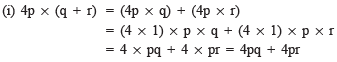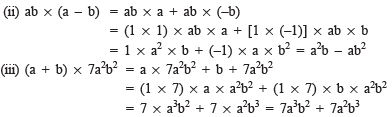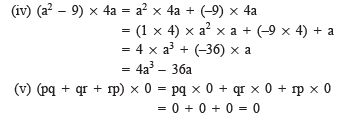Question 2. Complete the table.

 First expression Second expression Product (i) a b + c + d .... (ii) x + y - 5 5xy .... (iii) p 6p2 - 7p + 5 .... (iv) 4p2q2 p2 - q2 .... (v) a + b + c abc ....

Solution: We have: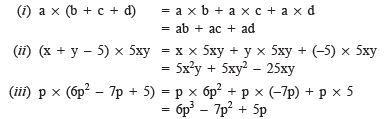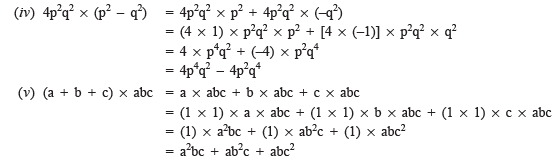Question 3. Find the product.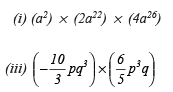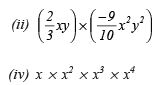Solution: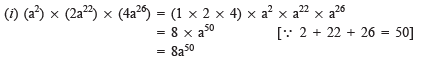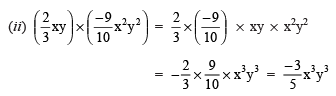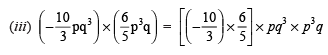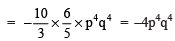(iv)  x*x2*x3*x4 = (1*1*1*1)*x*x2*x3*x4

= (1)*x10 = x10

Question 4. (a) Simplify 3x(4x – 5) + 3 and find its values for (i) x = 3 and (ii) x = 1/2 (b) Simplify a(a2 + a + 1) + 5 and find its value for (i) a = 0, (ii) a = 1 and (iii) a = –1.

Solution: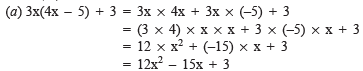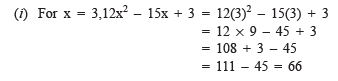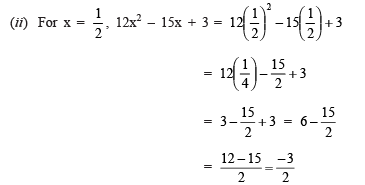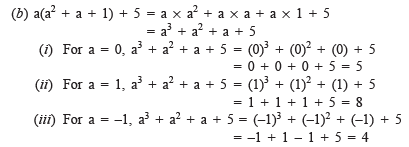Question 5.

(a) Add: p(p – q), q(q – r) and r(r – p)

(b) Add: 2x(z – x – y) and 2y(z – y – x)

(c) Subtract: 3l(l – 4m + 5n) from 41(10n + 3m + 2l)

(d) Subtract: 3a(a + b + c) – 2b(a – b + c) from 4c(–a + b + c)

Solution:

(a) ∵ p(p – q) = p* p – p* q = p2 – pq

q(q – r) = q* q – q* r = q2 – qr

and r(r – p) = r* r – r* p = r2 – rp

∴ Adding the above products, we have

(p2 – pq) + (q2 – qr) + (r2 – rp) = p2 – pq + q2 – qr + r2 – rp

= p2 + q2 + r2 – pq – qr – rp

= p2 + q2 + r2 – (pq + qr + rp)

(b) ∵ 2x(z – x – y) = 2x *z – 2x *x – 2x *y = 2xz – 2x2 – 2xy

and 2y(z – y – x) = 2y *z – 2y *y – 2y *x = 2yz – 2y2 – 2yx

∴  Adding the above products, we have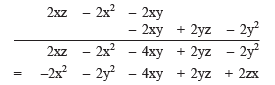(c) ∵ 3l(l – 4m + 5n) = 3l * l – 3l * 4m + 3l * 5n

= 3l2 – 12lm + 15ln

and 4l(10n – 3m + 2l)= 4l * 10n – 4l * 3m + 4l * 2l

= 40ln – 12lm + 8l2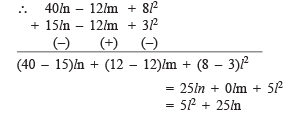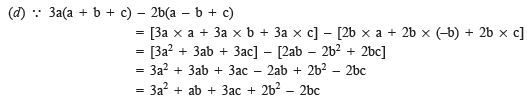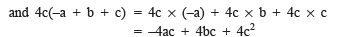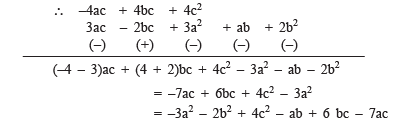Multiplying a Polynomial by a Polynomial

Remember

In multiplication of polynomials with polynomials, we always look for like terms, if any, and combine them.

Exercise 9.4

Question 1. Multiply the binomials.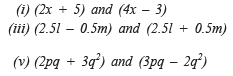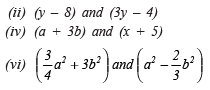Solution: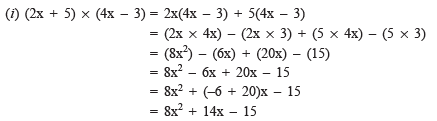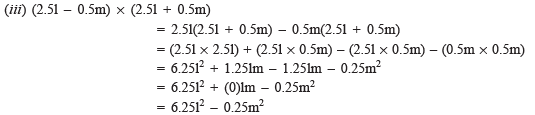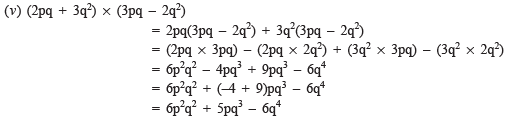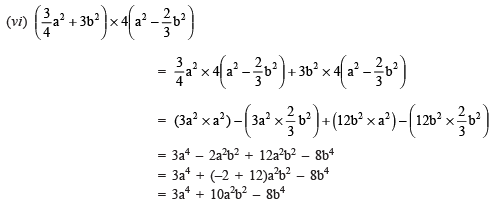Question 2. Find the product:

(i) (5 – 2x) (3 + x)  (ii) (x + 7y) (7x – y)
(iii) (a2 + b) (a + b2)  (iv) (p2 – q2)(2p + q)

Solution:

(i) (5 – 2x) * (x + 3) = 5(x + 3) – 2x(x + 3)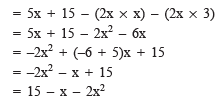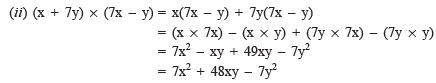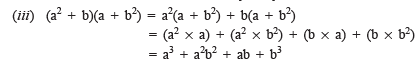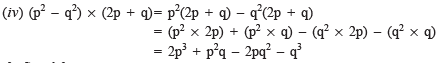Question 3. Simplify: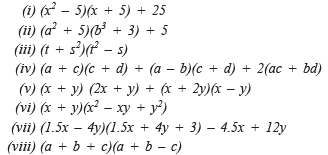Solution: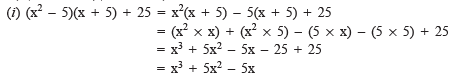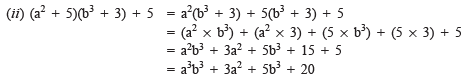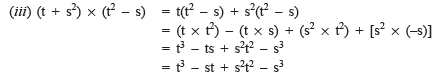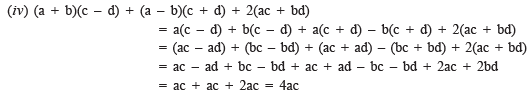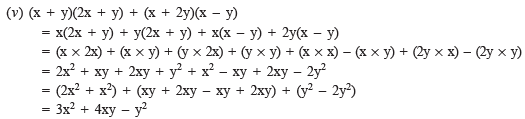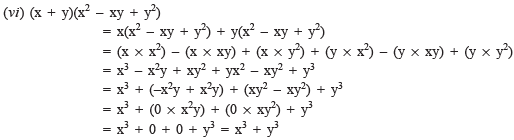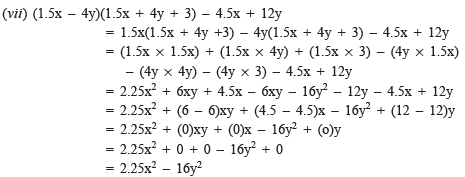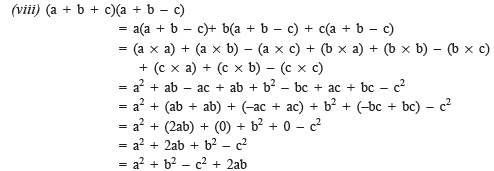Identities

We have studied equations earlier and we know that equations are true for certain values of the contained variables. That is the values of two sides (LHS and RHS) are equal only for certain values of variables, but it is not true for all values of the variable. If an equation is true for every value of the variable in it, then it is called an identity.

Standard Identities

Following equations are called the standard identities.

1. (a + b)2 = a2 + 2ab + b2
2. (a – b)2 = a2 – 2ab + b2
3. (a + b)(a – b) = a2 – b2
4. (x + a)(x + b) = x2 + (a + b)x + ab

Question 1. Verify Identity (IV), for a = 2, b = 3, x = 5

Solution: We have

(x + a)(x + b) = x2 + (a + b)x + ab

Putting a = 2, b = 3 and x = 5 in the identity:

LHS = (x + a)(x + b)

= (5 + 2)(5 + 3)

= 7 * 8 = 56

RHS = x2 + (a + b)x + ab

= (5)2 + (2 + 3) * 5 + (2 * 3)

= 25 + (5) * 5 + 6

= 25 + 25 + 6 = 56

∴ LHS = RHS

∴ The given identity is true for the given values.

Question 2. Consider the special case of Identity (IV) with a = b, what do you get? Is it related to Identity (I)?

Solution: When a = b (each = y)

(x + a)(x + b) = x2 + (a + b)x + ab becomes

(x + y)(x + y) = x2 + (y + y)x + (y* y)

= x2 + (2y)x + y2

= x2 + 2xy + y2

= Yes, it is the same as Identity I.

Question 3. Consider, the special case of Identity (IV) with a = –c and b = –c. What do you get? Is it related to Identity (II)?

Solution: Identity IV is given by

(x + a)(x + b) = x2 + (a + b)x + ab

Replacing ‘a’ by (–c) and ‘b’ by (–c), we have

(x – c)(x – c) = x2 + [(–c) + (–c)] x + [(–c) * (–c)]

= x2 + [–2c]x + (c2)

= x2 – 2cx + c2

Which is same as Identity II.

Question 4. Consider the special case of Identity (IV) with b = –a. What do you get? It is related to Identity (III).

Solution: The Identity IV is given by

(x + a)(x + b) = x2 + (a + b)x + ab

Replacing ‘b’ by (–a), we have:

(x + a)(x – a) = x2 + [a + (–a)]x + [a * (–a)]

= x2 + x + [–a2]

= x+ 0 + (–a2) = x2 – a2

Which is same as the Identity III.

Offer running on EduRev: Apply code STAYHOME200 to get INR 200 off on our premium plan EduRev Infinity!

93 docs|16 tests

,

,

,

,

,

,

,

,

,

,

,

,

,

,

,

,

,

,

,

,

,

;